Next: Numerical solution Up: The chaotic pendulum Previous: Introduction

## Analytic solution

Before attempting to solve the equations of motion of any dynamical system using a computer, we should, first, investigate them as thoroughly as possible via standard analytic techniques. Unfortunately, Eqs. (81) and (82) constitute a non-linear dynamical system--because of the presence of the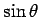term on the right-hand side of Eq. (82). This system, like most non-linear systems, does not possess a simple analytic solution. Fortunately, however, if we restrict our attention to small amplitude oscillations, such that the approximation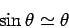(83)

is valid, then the system becomes linear, and can easily be solved analytically.

The linearized equations of motion of the pendulum take the form: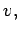(84)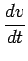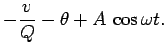(85)

Suppose that the pendulum's position,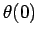, and velocity,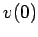, are specified at time. As is well-known, in this case, the above equations of motion can be solved analytically to give: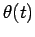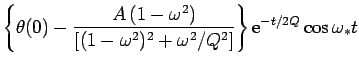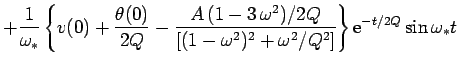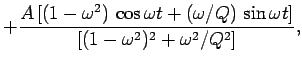(86)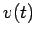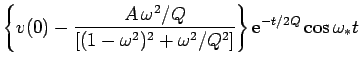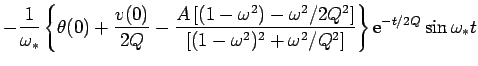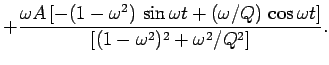(87)

Here,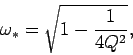(88)

and it is assumed that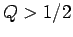. It can be seen that the above expressions forandboth consist of three terms. The first two terms clearly represent transients--they depend on the initial conditions, and decay exponentially in time. In fact, the e-folding time for the decay of these terms is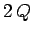(in normalized time units). The final term represents the time-asymptotic motion of the pendulum, and is manifestly independent of the initial conditions.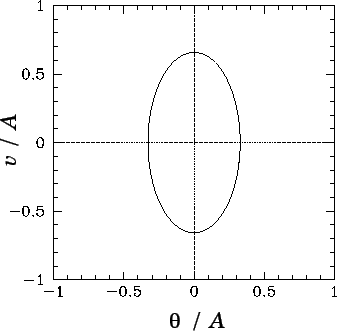It is often convenient to visualize the motion of a dynamical system as an orbit, or trajectory, in phase-space, which is defined as the space of all of the dynamical variables required to specify the instantaneous state of the system. For the case in hand, there are two dynamical variables,and, and so phase-space corresponds to the-plane. Note that each different point in this plane corresponds to a unique instantaneous state of the pendulum. [Strictly speaking, we should also considerto be a dynamical variable, since it appears explicitly on the right-hand side of Eq. (82).]

It is clear, from Eqs. (86) and (87), that if we wait long enough for all of the transients to decay away then the motion of the pendulum settles down to the following simple orbit in phase-space: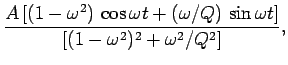(89)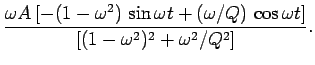(90)

This orbit traces out the closed curve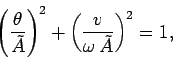(91)

in phase-space, where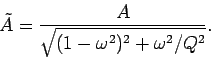(92)

As illustrated in Fig. 22, this curve is an ellipse whose principal axes are aligned with theandaxes. Observe that the curve is closed, which suggests that the associated motion is periodic in time. In fact, the motion repeats itself exactly every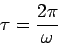(93)

normalized time units. The maximum angular displacement of the pendulum from its undriven rest position (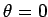) is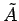. As illustrated in Fig. 23, the variation ofwith driving frequency[see Eq. (92)] displays all of the features of a classic resonance curve. The maximum amplitude of the driven oscillation is proportional to the quality-factor,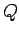, and is achieved when the driving frequency matches the natural frequency of the pendulum (i.e., when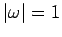). Moreover, the width of the resonance in-space is proportional to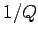.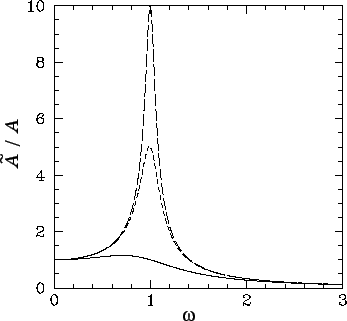The phase-space curve shown in Fig. 22 is called a periodic attractor. It is termed an attractor'' because, irrespective of the initial conditions, the trajectory of the system in phase-space tends asymptotically to--in other words, is attracted to--this curve as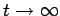. This gravitation of phase-space trajectories towards the attractor is illustrated in Figs. 24 and 25. Of course, the attractor is termed periodic'' because it corresponds to motion which is periodic in time.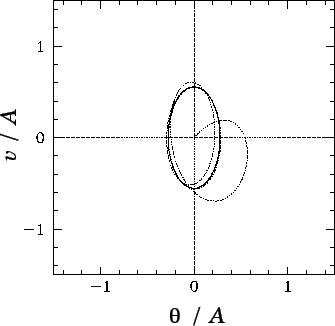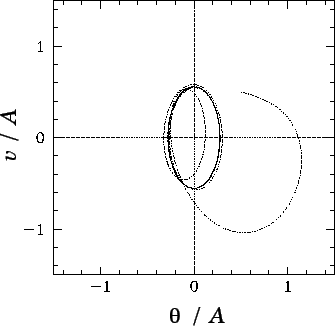Let us summarize our findings, so far. We have discovered that if a damped pendulum is subject to a low amplitude, periodic, drive then its time-asymptotic response (i.e., its response after any transients have died away) is periodic, with the same period as the driving torque. Moreover, the response exhibits resonant behaviour as the driving frequency approaches the natural frequency of oscillation of the pendulum. The amplitude of the resonant response, as well as the width of the resonant window, is governed by the amount of damping in the system. After a little reflection, we can easily appreciate that all of these results are a direct consequence of the linearity of the pendulum's equations of motion in the low amplitude limit. In fact, it is easily demonstrated that the time-asymptotic response of any intrinsically stable linear system (with a discrete spectrum of normal modes) to a periodic drive is periodic, with the same period as the drive. Moreover, if the driving frequency approaches one of the natural frequencies of oscillation of the system then the response exhibits resonant behaviour. But, is this the only allowable time-asymptotic response of a dynamical system to a periodic drive? Most undergraduate students might be forgiven for answering this question in the affirmative. After all, the majority of undergraduate classical dynamics courses focus almost exclusively on linear systems. The correct answer, as we shall see, is no. The response of a non-linear system to a periodic drive is generally far more rich and diverse than simple periodic motion. Since the majority of naturally occurring dynamical systems are non-linear, it is clearly important that we gain a basic understanding of this phenomenon. Unfortunately, we cannot achieve this goal via a standard analytic approach--non-linear equations of motion generally do not possess simple analytic solutions. Instead, we must use computers. As an example, let us investigate the dynamics of a damped pendulum, subject to a periodic drive, with no restrictions on the amplitude of the pendulum's motion.Next: Numerical solution Up: The chaotic pendulum Previous: Introduction
Richard Fitzpatrick 2006-03-29